# JP2004309285A - R/d converter - Google Patents

## Info

Publication number
JP2004309285A
JP2004309285A JP2003102568A JP2003102568A JP2004309285A JP 2004309285 A JP2004309285 A JP 2004309285A JP 2003102568 A JP2003102568 A JP 2003102568A JP 2003102568 A JP2003102568 A JP 2003102568A JP 2004309285 A JP2004309285 A JP 2004309285A
Authority
JP
Japan
Prior art keywords
θ
sine wave
value
output
wave value
Prior art date
Legal status (The legal status is an assumption and is not a legal conclusion. Google has not performed a legal analysis and makes no representation as to the accuracy of the status listed.)
Pending
Application number
JP2003102568A
Other languages
Japanese (ja)
Inventor
Takao Takehara

Original Assignee
Minebea Co Ltd
ミネベア株式会社
Priority date (The priority date is an assumption and is not a legal conclusion. Google has not performed a legal analysis and makes no representation as to the accuracy of the date listed.)
Filing date
Publication date
Application filed by Minebea Co Ltd, ミネベア株式会社 filed Critical Minebea Co Ltd
Priority to JP2003102568A priority Critical patent/JP2004309285A/en
Publication of JP2004309285A publication Critical patent/JP2004309285A/en
Pending legal-status Critical Current

• 238000006243 chemical reactions Methods 0.000 claims abstract description 28
• 230000000875 corresponding Effects 0.000 claims description 6
• 238000000034 methods Methods 0.000 description 2
• 230000000694 effects Effects 0.000 description 1

## Images

•••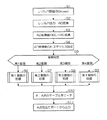•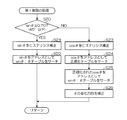•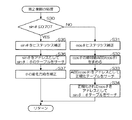•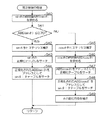•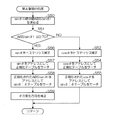•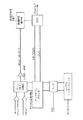## Abstract

An R / D converter capable of reducing an angle error and achieving a high-speed response is provided.
An AD converter (31) AD-converts a sine wave output and a cosine wave output of a resolver (1). The microcomputer 21 normalizes the above-mentioned AD conversion outputs sin θ and cos θ with the normalization table 35 according to the quadrant of the rotor axis, and performs hysteresis correction. Further, the rotor axis angle θ is read from the sin θ: θ table 34 using the normalized sin and cos θ corrected sin θ and cos θ as addresses, and the encode signals A and B are read from the θ: A and B tables using the rotor axis angle θ as the address. The data is read out and output from the output port 38.
[Selection diagram] Fig. 1

## Description


TECHNICAL FIELD OF THE INVENTION
The present invention relates to a converter for digitizing an output of a resolver (hereinafter, referred to as an R / D converter).

[Prior art]
The resolver is a type of rotary transformer, and includes two stator windings and one rotor winding. The two stator windings are mechanically offset by 90 degrees. The amplitude of the signal obtained by coupling with the stator winding is a function of the position of the rotor (axis) and the relative position of the stator. Therefore, the resolver outputs two types of output voltages (S3-S1, S4-) modulated by the sine (sine wave) and cosine (cosine wave) of the axis angle and represented by the following equations (1) and (2). S2) is obtained.
S3−S1 = A · sin ωt · sin θ (1)
S4−S2 = A · sin ωt · cos θ (2)
Here, θ: shaft angle, ω: angular velocity corresponding to the rotor excitation frequency (f), A: rotor excitation amplitude.

Conventionally, as an R / D converter, a technique for obtaining a resolver rotor shaft angle θ from the output voltages (S3-S1, S4-S2) with a circuit configuration shown in FIG. 8 has been proposed.

In the above prior art, when the transducer passes a position equal to the least significant bit, the output is updated by 1 LSB. Updating CLKOUT corresponds to an increase of 1 LSB. Assuming that the current word state of the up / down counter is φ, (S3-S1) is multiplied by cos φ by a sine cosine multiplier, and (S2-S4) is multiplied by sin φ, so that the following equation is obtained. Can be.
A · sinωt · sinθ · cosψ
A · sinωt · cosθ · sinｓ

When these signals are subtracted by the error amplifier, the following equation is obtained.
A · sinωt (sinθ · cosψ−cosθ · sinψ)

Or A · sin ωt · sin (θ-ψ)
Here, (θ−ψ) = angle error.

A closed loop is formed by a demodulation circuit, an integrator, and a voltage controlled oscillator (VCO), and the operation is performed so that sin (θ−ψ) becomes zero. When this operation is established, the word state の of the up / down counter becomes equal to the rotor shaft angle θ of the resolver.

[Problems to be solved by the invention]
However, in the above-described related art, since it is a kind of PLL control, there is a problem that the response speed is slow, and in particular, the response speed becomes slower as the resolution becomes higher. In addition, it is necessary to compensate for the temperature drift of the analog operation circuit, and there is a problem that the IC circuit becomes complicated and expensive. Further, when the interface cable between the resolver and the R / D converter becomes long, the phase shift becomes large due to the delay between the exciting sine wave and the sine wave output and cosine wave output from the resolver, and the angle error becomes large. is there.

The present invention has been made in view of the above circumstances, and has as its object to provide an R / D converter capable of reducing an angle error and achieving a high-speed response.

[Means for Solving the Problems]
The R / D converter according to the present invention converts the sine wave output and the cosine wave output of the resolver to AD in the R / D converter for obtaining the rotor shaft angle of the resolver based on the sine wave output and the cosine wave output of the resolver. An A / D converter; correction means for performing hysteresis correction on the sine wave value and the cosine wave value from the A / D converter; angle data storage means for holding rotor axis angle data of the resolver; Encoding data storage means for pre-holding encoding data corresponding to a value, and giving one of a sine wave value and a cosine wave value subjected to hysteresis correction by the correction means to the angle data storage means as an address, and providing a sine wave value and a cosine wave value. Angle data reading means for reading angle data corresponding to a value; Encoding data reading means for giving the angle data read by the stage as an address to the encoded data storage means and reading the encoded data; and output means for outputting the encoded data read by the encoded data reading means to the outside. It is characterized by having.

According to the R / D converter, the AD converter converts the sine wave output and the cosine wave output of the resolver to AD, and the correcting means corrects the AD converted sine wave value and cosine wave value in a hysteresis manner. The reading means gives one of the sine wave value and the cosine wave value subjected to the hysteresis correction to the angle data storage means as an address, reads out the angle data corresponding to the sine wave value and the cosine wave value, and further reads the encoded data. The angle data is given to the encoded data storage means as an address by the means, and the encoded data is read out and output from the output to the outside. Therefore, it is possible to reduce the angle error and to increase the response speed.

Further, in the present invention, a quadrant in which the rotor shaft of the resolver is formed by dividing the angular space of the resolver into four equal parts based on the polarities of the sine wave value and the cosine wave value from the AD converter by the correction means. It is preferable that hysteresis correction is performed on either the sine wave value or the cosine wave value from the AD converter based on the quadrant determined by the determination unit that determines which of the above.

In a preferred embodiment of the present invention, the normalization unit normalizes the sine wave value and the cosine wave value from the AD converter in order to correct the positive and negative asymmetry of the sine wave output and the cosine wave output of the resolver. I have. In a preferred embodiment of the present invention, the normalizing means normalizes a sine wave value and a cosine wave value in another quadrant based on a sine wave value in the first quadrant from the AD converter.

BEST MODE FOR CARRYING OUT THE INVENTION
Next, an embodiment of the present invention will be described with reference to the drawings.

A. First, FIG. 1 is a block diagram showing the configuration of an R / D converter according to one embodiment of the present invention. In FIG. 1, a resolver 1 is output from an R / D converter composed of a microcomputer to be described later, is excited by a sine wave for a resolver supplied via an LPF and an amplifier, and is rotated by a rotor directly connected to a rotating shaft such as a motor. A sine wave output and a cosine wave output linked to the rotation angle of the shaft are output.

The R / D converter 2 includes an amplifier 20, a microcomputer 21, an LPF (low-pass filter) 22, and an amplifier 23. The amplifier (AMP) 20 amplifies the sine wave output and the cosine wave output of the resolver 1 to a predetermined voltage, and supplies the amplified voltage to the microcomputer 21.

The microcomputer 21 includes an MPU 30, an AD converter 31, a DA converter 32, a data ROM 33, a sin θ: θ table 34, a normalization table 35, a θ: A, B table 36, and an output port 37. The sin θ: θ table 34, the normalization table 35, and the θ: A, B tables 36 may be provided individually or as one component as the ROM 37.

The AD converter 31 has, for example, a resolution of 10 to 14 bits, and performs AD conversion on the sine wave output and the cosine wave output of the resolver 1 from the amplifier 20 according to a predetermined sampling clock. On the other hand, the DA converter 32 converts a value read by DMA (direct memory access) from the data ROM 33 in which a value obtained by sampling a sine wave at a predetermined sampling frequency into an analog signal, and converts the value into an LPF 22 and an amplifier. The signal is supplied to the resolver 1 as a resolver excitation sine wave via the reference numeral 23. The LPF 22 removes a high-frequency component by quantizing the excitation sine wave to the resolver 1 output from the DA converter 32. The amplifier 23 amplifies the excitation sine wave from the LPF 22 and supplies the amplified sine wave to the resolver 1.

The MPU 30 synchronizes the sampling timing of the AD converter 31 and the timing of the DA converter 32 for generating a sine wave for excitation to the resolver 1. More specifically, data for the excitation signal is supplied to the DA converter 32 every 50 μsec. That is, in order to obtain a sine wave of 10 kHz, sine wave data of 20 samples is supplied per period. Further, the AD conversion in the AD converter 31 is performed every 10 kHz.

As described above, by synchronizing both, the sine wave output and the cosine wave output of the resolver 1 can be AD-converted at a specific phase of the excitation sine wave (positive or negative peak of the excitation sine wave), The above-mentioned sinωt and cosωt can be regarded as a constant “1”. As a result, the sine wave output and cosine wave output of the resolver can be set to sin θ and cos θ.

Further, in the MPU 30, the input signal from the amplifier 20 is a DC signal (sine wave) of 0 to 4 V for an input range of AD conversion of 0 to 5 V. -2V = AC sine wave. If the resolution of the AD conversion is 10 bits, then 2V = 1024 / 2.5 = 410 = 199H (hexadecimal). Therefore, the input signal is -199H = sine wave input of alternating current [sin θ (AC)].

Further, in the present embodiment, the AD conversion processing, the DA conversion processing, and the angle calculation processing are performed in synchronization with the timer interrupt. However, due to the overhead between the interrupts and noise to the AD converter input, the AD conversion value is reduced. An error occurs. In order to compensate for this, in the present embodiment, the AD conversion value (sin θ, cos θ) has a hysteresis characteristic. That is, when the difference between the current AD change value one sample before and the current value is within a certain value (for example, 5 LSB), the AD conversion value is set to the value one sample before. This can prevent chattering of the encoder pulse and pulse output when the motor is stopped.

FIG. 2 is a conceptual diagram showing sin θ and cos θ output from the AD converter 31 and the rotor shaft angle θ. In the present embodiment, the quadrant (current axis angle) of the resolver rotor is determined by dividing the 360 ° angular space of the rotor axis into four parts and checking the polarities of sinθ and cosθ of the AD conversion output. That is, as shown in FIG. 2, the 360-degree angle space is divided into four parts, the first quadrant between 0 and 90 degrees, the second quadrant between 90 and 180 degrees, and the third quadrant between 180 and 270 degrees. The fourth quadrant is defined as 360 °.

As can be seen from the drawing, the rotor shaft angle θ can be obtained by directly calculating from the above-mentioned sin θ and cos θ. However, the microcomputer 21 is slow in the calculation processing, so that the resolver 1 rotates at a high speed. Assuming that, it is difficult to obtain by direct calculation. Therefore, in the present embodiment, the rotor shaft angle θ is stored in advance in the range of 0 to 45 ° in the sin θ: θ table 34, and the stored rotor shaft angle θ is read out using the sin θ and cos θ as addresses. The rotor axis angle θ is obtained without performing advanced calculations. When the rotor shaft angle θ is 45 ° or more, sin θ / θ decreases, and the frequency of the encode signal decreases. Therefore, when the rotor shaft angle θ is 45 ° or less (that is, sin θ is 0.7707 or less), sin θ is is used as the address of the sin θ: θ table 34. When the angle is 45 ° or more (that is, sin θ is 0.7707 or more), cos θ is used as the address of the sin θ: θ table 34.

Further, the output of the resolver 1, that is, the amplitude of sin θ and the amplitude of cos θ are not the same, but are positive and negative asymmetric. Therefore, it is necessary to normalize cos θ to sin θ in the first quadrant. Assuming that the maximum values of sin θ and cos θ are sin θMAX and cos θMAX, respectively, the normalized value cos θN is cos θ × (sin θMAX / cos θMAX). More specifically, a COSθ normalization table 35 is prepared in advance using COSθ as an address, and the above sinθ is read from the normalization table 35 containing SINθ = COSθ × (SINθMAX / COSθMAX) using cosθ as an address. Is used to perform normalization. Even when sin θ is a negative value, normalization is performed in the same manner.

As described above, the MPU 30 performs the hysteresis processing and the normalization processing on the AD conversion values from the AD converter 31, that is, sin θ and cos θ, according to the quadrant where the rotor axis exists, and then obtains the rotor axis angle from the sin θ: θ table 34. is read out, and further, encode signals A and B are obtained from the rotor shaft angle θ and output from the output port 38. When calculating the encode signals A and B from the rotor shaft angle θ, instead of calculating, the θ: A, B table 36 in which the encode signals A and B are converted into data is prepared in advance, and the rotor shaft angle θ is used as an address. , Θ: A, B The encode signals A, B are obtained by reading the encode signals A, B from the table 36.

B. Next, an operation of the R / D converter according to the embodiment of the present invention will be described. Here, FIGS. 3 to 7 are flowcharts for explaining the operation of the R / D converter according to the present embodiment.

First, in step S1, the sampling frequency (SDFS) of the DA converter 32 is set to 200 KHz, and the value of the data ROM 33 is cyclically transmitted by circular addressing to generate an excitation sine wave of 200/20 = 10 KHz. , To the resolver via an amplifier. After the power is turned on, an initial excitation period of about 50 msec is provided, and the system stands by until the excitation state of the resolver is stabilized.

Next, in step S2, the sine wave output and the cosine wave output of the resolver 1 are fetched, and the AD converter 31 performs AD conversion. Here, the same signal is used as the sampling clock for the AD conversion and the DA conversion, and the sine wave output and the cosine wave output of the resolver 1 are AD-converted at a specific phase of the resolver excitation sine wave (positive or negative peak of the excitation sine wave). I do.

Next, in step S3, the DC-converted DC sin θ and cos θ are converted into AC sin θ and cos θ. Next, in step S4, the hysteresis (for example, 5LSB) of the AD conversion value is set. Next, in step S5, the current position (quadrant) of the rotor of the resolver 1 is determined by checking the polarities of sinθ and cosθ of the AD conversion output.

First, a case where the polarities of the sin conversion and cos θ of the AD conversion output are in the first quadrant (0 to 90 °) will be described. If the polarities of sin θ and cos θ are in the first quadrant, the process in the first quadrant is performed in step S6. In the processing in the first quadrant, first, in step S20, it is determined whether sin θ is 0.707 or less. If sin θ is 0.707 or less, that is, if the rotor shaft angle is 45 ° or less, hysteresis correction of sin θ is performed in step S21. That is, when the difference between the current sample θ and the current sin θ is within a certain value (5 LSB in this case), the value of sin θ is set to the value one sample before. Next, in step S22, the rotor shaft angle θ is read from the sin θ: θ table 34 using sin θ as an address. Next, returning to the routine of FIG. 3, in step S10, the encode signals A and B are read from the θ: A, B table 36 using the rotor shaft angle θ as an address, and the encode signals A and B are output in step S11. Output from port 38.

On the other hand, if sin θ is 0.707 or more in the first quadrant, that is, if sin θ is in the range of 45 ° to 90 °, hysteresis correction is performed on cos θ in step S23. That is, when the difference between the previous sample and the current cos θ is within a certain value (5LSB in this case), the value of cos θ is set to the value of the previous sample. Next, in step S24, the normalization table 35 for sin θ is searched and normalized using cos θ as an address. Next, in step S25, the rotor axis angle θ is read from the sin θ: θ table 34 using the normalized cos θ as an address. Next, in step S26, the rotor shaft angle θ is corrected by the equation θ = 45 ° −θ. This is because in the range of 45 ° to 90 °, instead of sin θ, cos θ, which decreases with an increase in the rotor axis angle, is used as an address. By this correction, the rotor axis angle becomes a value of 0 to 45 °. Take. Next, returning to the routine of FIG. 3, in step S10, the encode signals A and B are read from the θ: A, B table 36 using the rotor shaft angle θ as an address, and the encode signals A and B are output in step S11. Output from port 38.


On the other hand, in the second quadrant, if sin θ is 0.707 or less, that is, if the rotor shaft angle is in the range of 135 ° to 180 °, hysteresis correction of sin θ is performed in step S35. That is, when the difference between the current sample θ and the current sin θ is within a certain value (5 LSB in this case), the value of sin θ is set to the value one sample before. Next, in step S36, the rotor shaft angle θ is read from the sin θ: θ table 34 using sin θ as an address. Next, in step S37, the rotor shaft angle θ is corrected by the equation θ = 45 ° −θ. This is because sin θ decreases as the rotor axis angle θ increases in the range of 135 ° to 180 °, and the rotor axis angle takes a value of 0 to 45 ° by this correction. Next, returning to the routine of FIG. 3, in step S10, the encode signals A and B are read from the θ: A, B table 36 using the rotor shaft angle θ as an address, and the encode signals A and B are output in step S11. Output from port 38.


On the other hand, if sin θ is 0.707 or more in the third quadrant, that is, if sin θ is in the range of 225 ° to 270 °, hysteresis correction is performed on cos θ in step S45. That is, when the difference between the previous sample and the current cos θ is within a certain value (5LSB in this case), the value of cos θ is set to the value of the previous sample. Next, in step S46, an absolute value ABS (cos θ) of cos θ is obtained. This is because cos θ is negative in the third quadrant. Next, in step S47, the normalization table 35 for sin θ is searched and normalized using cos θ as an address. Next, in step S48, the rotor axis angle θ is read from the sin θ: θ table 34 using the normalized cos θ as an address. Next, in step S49, the rotor shaft angle θ is corrected by the equation θ = 45 ° −θ. This is because in the range of 225 ° to 270 °, instead of sin θ, cos θ, which decreases with an increase in the rotor axis angle, is used as an address. By this correction, the rotor axis angle becomes a value of 0 to 45 °. Take. Next, returning to the routine of FIG. 3, in step S10, the encode signals A and B are read from the θ: A, B table 36 using the rotor shaft angle θ as an address, and the encode signals A and B are output in step S11. Output from port 38.


On the other hand, if sin θ is 0.707 or less in the fourth quadrant, that is, if the rotor shaft angle is in the range of 315 ° to 360 °, hysteresis correction of sin θ is performed in step S56. That is, when the difference between the current sample θ and the current sin θ is within a certain value (5 LSB in this case), the value of sin θ is set to the value one sample before. Next, in step S57, the normalization table 35 for sinθ is searched and normalized. This is because sin θ is negative (asymmetric with respect to sin θ in the first quadrant) in the fourth quadrant. Next, in step S58, the rotor shaft angle θ is read from the sin θ: θ table 34 using the absolute value ABS (sin θ) of sin θ as an address. Next, in step S59, the rotor shaft angle θ is corrected by the equation θ = 45 ° −θ. This is because in the range of 315 ° to 360 °, sin θ decreases as the angle θ increases, and the rotor shaft angle takes a value of 0 to 45 ° by this correction. Next, returning to the routine of FIG. 3, in step S10, the encode signals A and B are read from the θ: A, B table 36 using the rotor shaft angle θ as an address, and the encode signals A and B are output in step S11. Output from port 38.

As described above, according to the present embodiment, since a high-level operation is not required, even the microcomputer 21 having a low operation capability can sufficiently cope with the high rotation of the resolver 1. In addition, by giving the AD conversion value a hysteresis characteristic, it is possible to compensate for an error generated in the AD conversion value due to an overhead between interrupts, noise to an AD converter input, and the like. Also, by normalizing the outputs sin θ and cos θ of the resolver 1, an angle error due to positive / negative asymmetry can be corrected.

【The invention's effect】
[Brief description of the drawings]
FIG. 1 is a block diagram illustrating a configuration of an R / D converter according to an embodiment of the present invention.
FIG. 2 is a conceptual diagram showing an angle and a quadrant of a resolver.
FIG. 3 is a flowchart for explaining the operation of the R / D converter according to the embodiment;
FIG. 4 is a flowchart for explaining the operation of the R / D converter according to the embodiment;
FIG. 5 is a flowchart for explaining the operation of the R / D converter according to the embodiment;
FIG. 6 is a flowchart for explaining the operation of the R / D converter according to the embodiment;
FIG. 7 is a flowchart for explaining the operation of the R / D converter according to the embodiment;
FIG. 8 is a block diagram showing a configuration of a conventional R / D converter.
[Explanation of symbols]
1 Resolver 2 R / D converter 21 Microcomputer 22 LPF
23 amplifier 30 MPU (correction means, angle data reading means, encoded data reading means, judgment means)
31 AD converter 32 DA converter 33 Data ROM
34 sin θ: θ table (angle data storage means)
35 Normalization table (normalization means)
36 θ: A, B table (encoding data storage means)
38 Output port (output means)

## Claims (4)

1. An R / D converter for determining a rotor axis angle of the resolver based on a sine wave output and a cosine wave output of the resolver,
An AD converter that performs AD conversion on a sine wave output and a cosine wave output of the resolver;
Correction means for performing hysteresis correction on the sine wave value and the cosine wave value from the AD converter;
Angle data storage means for holding the rotor axis angle data of the resolver,
Encoding data storage means for previously holding encoding data for the sine wave value and the cosine wave value from the AD converter;
Angle data reading means for giving one of a sine wave value and a cosine wave value subjected to hysteresis correction by the correction means as an address to the angle data storage means, and reading angle data corresponding to the sine wave value and the cosine wave value,
Encoding data reading means for giving the angle data read by the angle data reading means to the encoded data storage means as an address, and reading the encoded data;
Output means for outputting the encoded data read by the encoded data reading means to the outside.
2. Determining means for determining which of a quadrant formed by dividing the angular space of the resolver into four equal parts based on the polarity of the sine wave value and the cosine wave value from the AD converter; Equipped,
The correction means,
2. The R / D converter according to claim 1, wherein one of a sine wave value and a cosine wave value from the AD converter is subjected to hysteresis correction based on the quadrant determined by the determination unit.
3. 2. The apparatus according to claim 1, further comprising a normalizing unit that normalizes a sine wave value and a cosine wave value from the AD converter to correct positive / negative asymmetry of the sine wave output and the cosine wave output of the resolver. R / D converter.
4. 4. The R / D converter according to claim 3, wherein the normalizing means normalizes a sine wave value and a cosine wave value in another quadrant based on the sine wave value in the first quadrant from the AD converter.
JP2003102568A 2003-04-07 2003-04-07 R/d converter Pending JP2004309285A (en)

## Priority Applications (1)

Application Number Priority Date Filing Date Title
JP2003102568A JP2004309285A (en) 2003-04-07 2003-04-07 R/d converter

## Applications Claiming Priority (1)

Application Number Priority Date Filing Date Title
JP2003102568A JP2004309285A (en) 2003-04-07 2003-04-07 R/d converter

## Publications (1)

Publication Number Publication Date
JP2004309285A true JP2004309285A (en) 2004-11-04

# Family

## Family Applications (1)

Application Number Title Priority Date Filing Date
JP2003102568A Pending JP2004309285A (en) 2003-04-07 2003-04-07 R/d converter

## Country Status (1)

JP (1) JP2004309285A (en)

## Cited By (5)

* Cited by examiner, † Cited by third party
Publication number Priority date Publication date Assignee Title
JP2007248246A (en) * 2006-03-15 2007-09-27 Omron Corp Resolver signal processing device
JP2010022112A (en) * 2008-07-09 2010-01-28 Mitsubishi Electric Corp Temperature detection device of brushless motor
JP2011257254A (en) * 2010-06-09 2011-12-22 Hitachi Automotive Systems Ltd Rotation angle detection device
DE102015102370A1 (en) 2014-03-26 2015-10-01 Toyota Jidosha Kabushiki Kaisha Resolver excitation circuit
EP3054598A1 (en) 2015-01-29 2016-08-10 Renesas Electronics Corporation A resolver-to-digital converter with a lower error of the conversion angle

## Cited By (7)

* Cited by examiner, † Cited by third party
Publication number Priority date Publication date Assignee Title
JP2007248246A (en) * 2006-03-15 2007-09-27 Omron Corp Resolver signal processing device
JP2010022112A (en) * 2008-07-09 2010-01-28 Mitsubishi Electric Corp Temperature detection device of brushless motor
JP2011257254A (en) * 2010-06-09 2011-12-22 Hitachi Automotive Systems Ltd Rotation angle detection device
DE102015102370A1 (en) 2014-03-26 2015-10-01 Toyota Jidosha Kabushiki Kaisha Resolver excitation circuit
EP3054598A1 (en) 2015-01-29 2016-08-10 Renesas Electronics Corporation A resolver-to-digital converter with a lower error of the conversion angle
US10033397B2 (en) 2015-01-29 2018-07-24 Renesas Electronics Corporation Semiconductor device
US10530383B2 (en) 2015-01-29 2020-01-07 Renesas Electronics Corporation Semiconductor device

## Similar Documents

Publication Publication Date Title
US9463713B2 (en) System and method for error correction in angular position sensors
US7664619B2 (en) Fault detection unit for rotation angle detecting device
EP1746392B1 (en) Phase detection circuit, resolver/digital converter using the circuit, method performing digital conversion of an analog signal, and control system using the converter
DE19502399C2 (en) Method for correcting errors in a position sensor
Bergas-Jané et al. High-accuracy all-digital resolver-to-digital conversion
KR20190002756A (en) Position estimation method, position estimation device and position control device
US5796231A (en) Rotation position detecting device and motor device
US5162798A (en) Resolver to digital converter
JP5592270B2 (en) Method and apparatus for angle sensor
CN102472640B (en) Resolver signal conversion device and method
US7123175B2 (en) Angle detection apparatus computing phase differences between first and second phase angles
DE69932980T2 (en) resolver
EP1425855B1 (en) A wide band digital phase locked loop (pll) with a half-frequency output
JP2013253988A (en) Method and apparatus for analog rotary sensor
US6522097B2 (en) Position detecting apparatus and abnormality detecting apparatus
DE69634656T2 (en) Device and method for phase detection for a position detection system
AU729551B2 (en) A digital control system for a vibrating structure gyroscope
US5646496A (en) Apparatus and method for generating digital position signals for a rotatable shaft
TWI646308B (en) The signals from the gyroscope circuit for processing
JP6163874B2 (en) Rotation angle detection device, image processing device, and rotation angle detection method
US6084376A (en) Low cost resolver system
US20100156397A1 (en) Methods and apparatus for an angle sensor for a through shaft
US5949359A (en) Variable reluctance resolver to digital converter
EP1722193B1 (en) Hemispherical resonator gyro control
CA1270542A (en) Method and apparatus for correcting resolver errors

## Legal Events

Date Code Title Description
A621 Written request for application examination

Free format text: JAPANESE INTERMEDIATE CODE: A621

Effective date: 20041124

A977 Report on retrieval

Effective date: 20061222

Free format text: JAPANESE INTERMEDIATE CODE: A971007

A131 Notification of reasons for refusal

Free format text: JAPANESE INTERMEDIATE CODE: A131

Effective date: 20061228

A521 Written amendment

Effective date: 20070202

Free format text: JAPANESE INTERMEDIATE CODE: A523

A131 Notification of reasons for refusal

Free format text: JAPANESE INTERMEDIATE CODE: A131

Effective date: 20080718

A02 Decision of refusal

Effective date: 20081107

Free format text: JAPANESE INTERMEDIATE CODE: A02